## Ohm's Law Resistance and Resistors

Ohm’s Law Resistance and Resistors

Resistance is the difficulty applied by the conductor to the current flowing through it. Each material has different resistance. We show resistance with R and unit of it is ohm (Ω).

1 Ω=resistance of the conductor when 1 A current flows under the 1 V potential difference. Resistance is represented with the following picture in circuits;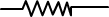Rheostat

Rheostat is a kind of device used to vary existing resistance. It is shown in the circuits as;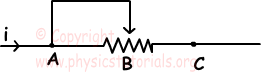Resistance of the conductor depends on;

· Types of the material or electrical resistivity of the material. It is shown with Greek letter ρ. Resistance of the material is linearly proportional to electrical resistivity.

· Length of the material (l). Resistance is linearly proportional to the length of the conductor.

· Cross sectional are of the conductor. Resistance is inversely proportional to the cross sectional area.

· Temperature. Temperature shows different effects with respect to the type of material.

We write resistance formula with the explanation given above as;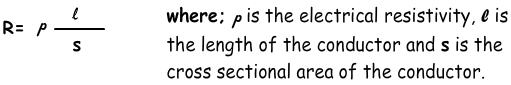Ohm’s Law

Ohm’s law gives the relation between voltage, current and resistance. According to Ohm, current in a circuit is directly proportional to the applied voltage and inversely proportional to the resistance of the conductor. We can summarize this explanation with following formula;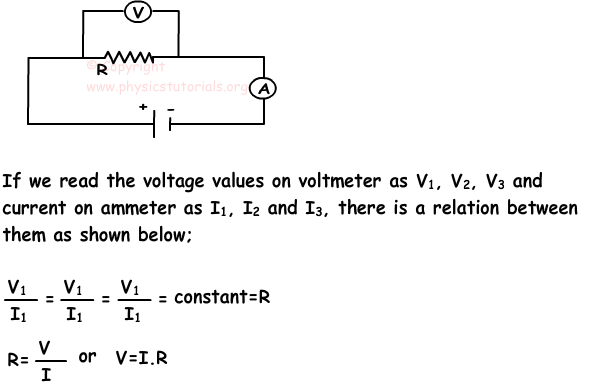If we take resistance of the conductor constant, then potential difference and current of the system changes linearly as shown in the following graph;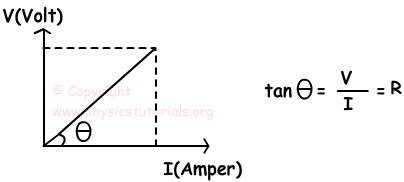Example: Potential difference vs. Current graph of a conductor is given below, Find the behavior of resistance in intervals I, II and III.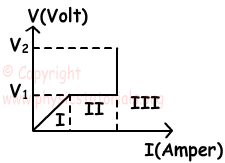Ohm’s law states that V=I.R

• In the first interval, since the potential difference and current are linearly increases then resistance of the system becomes constant.
• In the second interval, potential difference is constant however, current increases. This can be possible by decreasing in the amount of resistance.
• In the third interval, current is constant, however, potential difference increases. This can be possible by increasing in the amount of resistance.

Electric Current Exams and Solutions

Author: